# AP Calculus AB Practice Test 2

### Test Information10 questions20 minutes

Calculator Disallowed

1. If f(x) =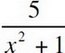and g(x) = 3x, then g(f(2)) =

2.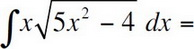3. The slope of the line tangent to the graph of 3x2 + 5 ln y = 12 at (2, 1) is

4. The equation y = 2 - 3 sin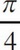(x - 1) has a fundamental period of

5. If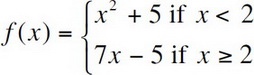, for all real numbers x, which of the following must be true?

I. f(x) is continuous everywhere.

II. f(x) is differentiable everywhere.

III. f(x) has a local minimum at x = 2.

6. For what value of x does the function f(x) = x3 - 9x2 - 120x + 6 have a local minimum?

7. The acceleration of a particle moving along the x-axis at time t is given by a(t) = 4t - 12. If the velocity is 10 when t = 0 and the position is 4 when t = 0, then the particle is changing direction at

8. The average value of the function f(x) = (x -1)2 on the interval from x = 1 to x = 5 is

9.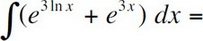10. If f(x) = (x2 + x + 11)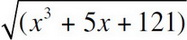, then f′(0) =